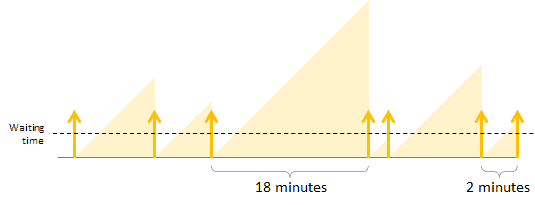# The next block is 10 minutes away: Proving bitcoin’s poisson block intervals

### Contents

Because there is a bitcoin block every 10 minutes on average, I assumed if I made a transaction it would get 1 confirmation 5 minutes later on average.

It seems like reasonable common sense and logic that with an average block interval of 10 minutes, any random time will be on average 5 minutes until the next block. Here is why I was wrong and the real world bitcoin data to prove it.

## You’re more likely to make a transaction in a long block interval than in a short block interval

If there was a new block exactly every 10 minutes, then the average interval between a random time and and the next block would be 5 minutes.

However in bitcoin the time between blocks is not regular. It varies greatly, from 0 minutes up to… well in practice the longest block interval is 1508 minutes (not counting block 0 to block 1).

That means a random time might fall in a shorter interval of less than 10 minutes, or a longer interval of more than 10 minutes. It’s more likely to fall in an interval of more than 10 minutes, simply because those intervals last longer.

This is easy to understand with a small scale example. Consider a 20 minute timespan that includes a 2 minute block interval and an 18 minute block interval. Now think of a random time in that 20 minute timespan. Is the random time more likely to fall in the 2 minute block interval or in the 18 minute block interval? It’s 9 times more likely to fall in the 18 minute block interval because that interval lasts 9 times longer!A random time is more likely to fall in the 18 minute interval than the 2 minute interval.

In probability terminology this is because bitcoin block intervals follow a poisson distribution.

## Real world proof with bitcoin block data

There is no need to take anyone’s word for it. I have proven that bitcoin blocks follow a poisson distribution and that on average the next block is 10 minutes away from any random time, such as the time you made your transaction.

First I downloaded the block timestamps from the 15th of March 2018 to the 15th of March 2019. That gave me 53,542 block times. Then I got 9968 random datetimes from within that timespan. All the datetimes were in the format

`yyyy-mm-dd hh:mm:ss`

For example

`2018-03-15 10:03:26`

In order to convert those to unix epochs I used the spreadsheet formula

`SUM=(A5-DATE(1970,1,1))*86400`

Where A5 is the cell containing the datetime I want to convert.

Unix epoch is the number of seconds since 1970. This makes it easy to calculate the number of seconds difference between datetimes.

The final step was calculating the interval between each random datetime and the next block. I did that by combining the block datetimes and the random datetimes in a single column ordered by time, with the random datetimes identifiable by color. Lastly subtracting the unix epoch of the random datetime from the unix epoch of the next block gave me the number of seconds between them.

The average is 9 minutes and 38 seconds. Exactly what the poisson distribution theory predicts! All of this can be seen, downloaded and played with on this google sheet (there are 2 tabs).

Of course this applies to other blockchains with variable block intervals. For example, litecoin and dash have 2.5 minute block intervals, so the average time from when you made your transaction until it gets 1 confirmation is 2.5 minutes.

## Why its a bit less than 10 exactly

In theory there is a bitcoin block found, on average, every 10 minutes. In reality it will tend to be slightly shorter than that in a difficulty period in which network hashrate increases, and it will tend to be slightly longer than that in a difficulty period in which network hashrate decreases.

Bitcoin miners have to solve math problems to find blocks. The more computing power they have, the faster they will solve the problems and find new blocks.

If miners find blocks faster than 1 every 10 minutes, then the bitcoin difficulty adjustment will make it harder for them, but that only happens every 2 weeks (2016 blocks). If the amount of computing power miners have increases in the first week of a difficulty period, they will have another week to find blocks faster than 1 every 10 minutes before the difficulty adjusts again.

The amount of computing power miners use is measured by hashrate. In March 2018 the bitcoin network performed around 25 million terahashes per second. By March 2019 it was around 43 million terahashes per second.

So during the timespan of this data the miners computing power was increasing, allowing them to find blocks slightly faster than every 10 minutes. During the timespan of this data a block was found every 9:49. The average interval between a random time and the next block was 9:38. Only 11 seconds apart or 1.87% apart.

```(578/589)*100 = 98.13% similarity
100-98.13 = 1.87% difference```

## Tools and resources used

### 1 comment

1.#### Marcus

Could a bitcoin block be found in say 5 minutes? Surely it’s possible, just highly unlikely?

EXCLUSIVE BONUSES

### Only on BitEdge!

• Free bets
• Free spins
• Free rolls
• Deposit matches
Get crypto bonuses